### DIFFERENTIAL GEOMETRY COURSE

1. VECTORS

Definition 1: Consider points X, Y, ... in space. Let XY be the directed line segment from X to Y. (We call X the tail and Y the head). So this line segment has a length and a direction (except if X=Y).
We call two such directed line segments equivalent if they have the same length and the same direction, or if they have both length 0. We call each equivalence class a vector (and the class of the line segments with length 0 is called the zero vector, 0).

Now let O be a fixed point in space (, called origin). We associate each point X with a unique vector x with representative OX. We call OX the main representative of x, and x the place vector of X.

The length of a vector x, which we denote by ||x||, is the length of any of its representatives.

Definition 2: If a is a vector and λ a real number, then λa is the vector with ||λa|| = |λ| ||a||, having the same direction as a if λ > 0, and the opposite direction if λ < 0.

We add vectors using the head-on-tail method (rule of parallelograms)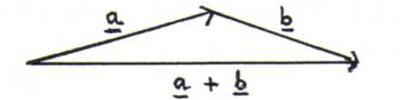Note 3:
(vector representation of a straight line)
The vectors sv (λ ∈ ℜ) are place vectors of points on a straight line. (We call s supporting vector and v direction vector of this line.)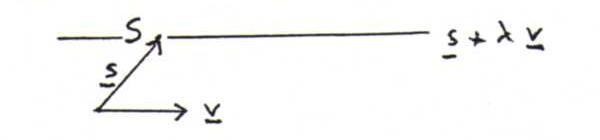Whenever A and B are arbitrary points, the place vectors of the points on the line through A and B all have the form a+λ(b-a).
Check the following: XY+YZ=XZ, so AB=OB-OA=b-a.

(coordinates in a plane)
Suppose that O, A and B don't lie on a (straight) line. Let X be an arbitrary point in the plane α door O, A and B. Then we can uniquely write X in the form λab for certain real numbers λ and μ; we call λ and μ coordinates of x with respect to base a, b.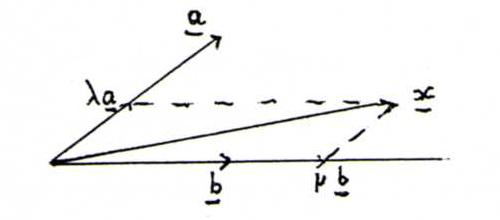(vector representation of a plane)
In general, the vectors svw are place vectors of points in a plane.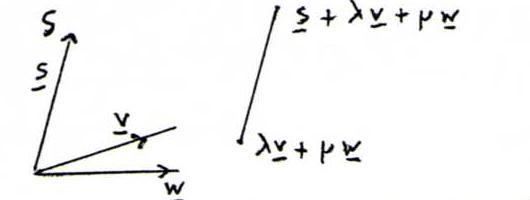The place vectors of the points in the plane through three non-collinear points A, B and C all have the form a+λ(b-a)+μ(c-a).

(coordinates in space)
Suppose that O, A, B don't lie C in one plane. Let X be an arbitrary point in space. Then we can uniquely write x in the form λabc for certain real numbers λ, μ and ν (coordinates of x with respect to base a, b, c).
If a, b and c have length 1 and each pair out of them is perpendicular, we call the base orthonormal.

(natural coordinates)
We start with a fixed orthonormal base e1, e2, e3, where e3 is chosen with e1 and e2 according to the corkscrew rule. We denote x=x1e1+x2e2+x3e3 by x=(x1,x2,x3).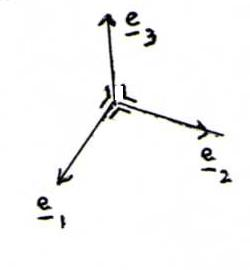Let x=(x1,x2,x3) and y=(y1,y2,y3). Then, for each real number λ, λx= (λx1,λx2,λx3) and x+y=(x1+y1,x2+y2,x3+y3).
According to Pythagoras, the distance between x and y is equal to ||x-y||=√((x1-y1)2+(x2-y2)2+(x3-y3)2).

Definition 4: The inner product (scalar product) of x and y is the number x.y := x1y1+x2y2+x3y3.
The outer product (vector product) of x and y is the vector xy := (x2y3-x3y2,x3y1-x1y3,x1y2-x2y1).

Problem 5:Prove by using the cosine rule (from triangular geometry) that x.y=||x||.||y||.cos(φ), where φ is the angle between x and y.
Using the inner product, prove that xy is perpendicular to x and y.

Note 6: (equations of a plane)
Let n be an arbitrary vector, not equal to 0. The points X whose place vectors satisfy x.n=0 are lying in the plane through 0 perpendicular to n. Eeach plane with equation x.n=c (with c∈ℜ) is parallel to this plane.

Problem 7: Prove the identities det(a,b,c)=ab.c and a⊗(bc)=(a.c)b-(a.b)c.
Thereafter, prove that det(x-a,b-a,c-a)=0 and (x-a).((b-a)⊗(c-a))=0 are equations of the plane through A, B and C.

Problem 8: Suppose b1, b2, b3 is an orthonormal base, and x1b12b23b3. Prove that x.bii.

Problem 9: Let x(t)=(x1(t),x2(t),x3(t)), where x1,x2 and x3 are differentiable functions of a real parameter t that runs through an interval I. Analogously, let y(t)=(y1(t),y2(t),y3(t)).
Denote xi'(t) := (d/dt) xi(t) and x'(t)=(x1'(t),x2'(t),x3'(t)), etc.
Prove:
a) (d/dt) x(t).y(t) = x'(t).y(t)+x(t).y'(t).
b) If ||x(t)||=1 for t∈I, then x(t) is perpendicular to x'(t) for all t∈I.
c) (d/dt) x(t)⊗y(t) = x'(t)⊗y(t)+x(t)⊗y'(t).